# AP Physics C: Electricity and Magnetism Practice Test 2

### Test Information10 questions13 minutes

1. Each of the resistors shown in the circuit below has a resistance of 200 Ω. The emf of the ideal battery is 24 V.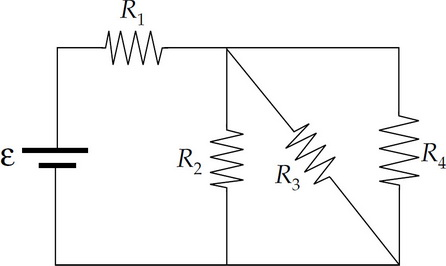What is the ratio of the power dissipated by R1 to the power dissipated by R4?

2. What is the value of the following product?

20 μF × 500 Ω

3.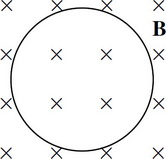A copper wire in the shape of a circle of radius 1 m, lying in the plane of the page, is immersed in a magnetic field, B, that points into the plane of the page. The strength of B varies with time, t, according to the equation

B(t) = 2t(1 – t)

where B is given in teslas when t is measured in seconds. What is the magnitude of the induced electric field in the wire at time t = 1 s?

4.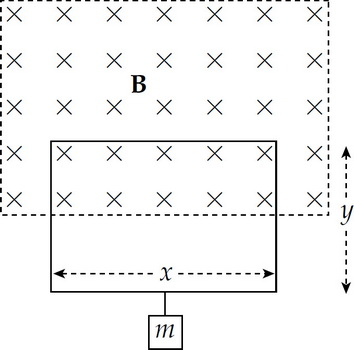In the figure above, the top half of a rectangular loop of wire, x meters by y meters, hangs vertically in a uniform magnetic field, B. Describe the magnitude and direction of the current in the loop necessary for the magnetic force to balance the weight of the mass m supported by the loop.

5.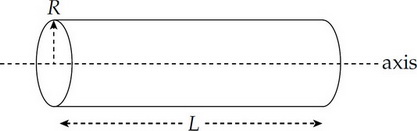A solid nonconducting cylinder of radius R and length L contains a volume charge density given by the equation ρ(r) = (+3 C/m4)r, where r is the radial distance from the cylinder's central axis. This means that the total charge contained within a concentric cylinder of radius r < R and length 这是啥 < L is equal to 2π这是啥r3. Find an expression for the strength of the electric field inside this cylinder.

6.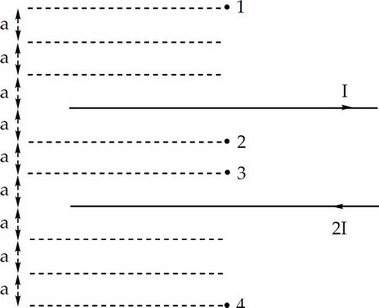The figure above shows a pair of long, straight current-carrying wires and four marked points. At which of these points is the net magnetic field zero?

7.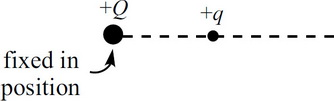The figure above shows two positively charged particles. The +Q charge is fixed in position, and the +q charge is brought close to +Q and released from rest. Which of the following graphs best depicts the acceleration of the +q charge as a function of its distance r from +Q?

8.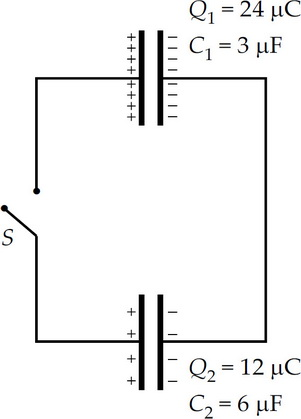Once the switch S in the figure above is closed and electrostatic equilibrium is regained, how much charge will be stored on the positive plate of the 6 μF capacitor?

9.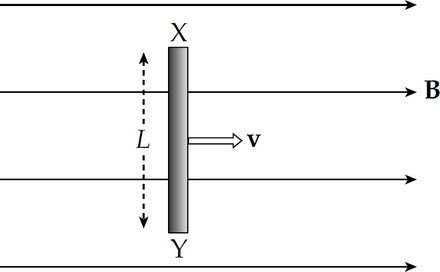A metal bar of length L is pulled with velocity v through a uniform magnetic field, B, as shown above. What is the voltage produced between the ends of the bar?

10. An electric dipole consists of a pair of equal but opposite point charges of magnitude 4.0 nC separated by a distance of 2.0 cm. What is the electric field strength at the point midway between the charges?